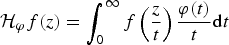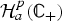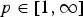Hostname: page-component-797576ffbb-jhnrh Total loading time: 0 Render date: 2023-12-09T03:13:50.927Z Has data issue: false Feature Flags: { "corePageComponentGetUserInfoFromSharedSession": true, "coreDisableEcommerce": false, "useRatesEcommerce": true } hasContentIssue false

# Hausdorff operators on holomorphic Hardy spaces and applications

Published online by Cambridge University Press:  30 January 2019

## Abstract

The aim of this paper is to characterize the non-negative functions φ defined on (0,∞) for which the Hausdorff operator$${\rm {\cal H}}_\varphi f(z) = \int_0^\infty f \left( {\displaystyle{z \over t}} \right)\displaystyle{{\varphi (t)} \over t}{\rm d}t$$
is bounded on the Hardy spaces of the upper half-plane${\rm {\cal H}}_a^p ({\open C}_ + )$,$p\in [1,\infty ]$. The corresponding operator norms and their applications are also given.

Type
Research Article

## Access options

Get access to the full version of this content by using one of the access options below. (Log in options will check for institutional or personal access. Content may require purchase if you do not have access.)

## References

1Andersen, K. F.. Boundedness of Hausdorff operators on L p(ℝn), H 1(ℝn), and BMO(ℝn). Acta Sci. Math. (Szeged) 69 (2003), 409418.Google Scholar
2Arvanitidis, A. G. and Siskakis, A. G.. Cesàro operators on the Hardy spaces of the half-plane. Can. Math. Bull. 56 (2013), 229240.CrossRefGoogle Scholar
3Ballamoole, S., Bonyo, J. O., Miller, T. L. and Miller, V. G.. Cesàro-like operators on the Hardy and Bergman spaces of the half plane. Complex Anal. Oper. Theory 10 (2016), 187203.CrossRefGoogle Scholar
4Brown, A., Halmos, P. and Shields, A.. Cesàro operators. Acta Sci. Math. (Szeged) 26 (1965), 125137.Google Scholar
5Carro, M. J. and Ortiz-Caraballo, C.. Boundedness of integral operators on decreasing functions. Proc. R. Soc. Edinburgh Sect. A 145 (2015), 725744.CrossRefGoogle Scholar
6Duren, P. L.. Theory of H p spaces: pure and applied mathematics, vol. 38 (New York-London: Academic Press, 1970).Google Scholar
7Fefferman, C. and Stein, E. M.. H p spaces of several variables. Acta Math. 129 (1972), 137193.CrossRefGoogle Scholar
8Galanopoulos, P. and Papadimitrakis, M.. Hausdorff and quasi-Hausdorff matrices on spaces of analytic functions. Can. J. Math., 58 (2006), 548579.CrossRefGoogle Scholar
9Garnett, J. B.. Bounded analytic functions. Graduate texts in Mathematics, Revised 1st edn, vol. 236 (New York: Springer, 2007).Google Scholar
10Hung, H. D., Ky, L. D. and Quang, T. T.. Norm of the Hausdorff operator on the real Hardy space H 1(ℝ). Complex Anal. Oper. Theory 12 (2018), 235245.CrossRefGoogle Scholar
11John, F. and Nirenberg, L.. On functions of bounded mean oscillation. Comm. Pure Appl. Math. 14 (1961), 415426.CrossRefGoogle Scholar
12Lerner, A. K. and Liflyand, E.. Multidimensional Hausdorff operators on the real Hardy space. J. Aust. Math. Soc. 83 (2007), 7986.CrossRefGoogle Scholar
13Liflyand, E.. Open problems on Hausdorff operators. Complex analysis and potential theory,pp. 280285 (Hackensack, NJ: World Sci. Publ., 2007).CrossRefGoogle Scholar
14Liflyand, E.. Boundedness of multidimensional Hausdorff operators on H 1(ℝn). Acta Sci. Math. (Szeged). 74 (2008), 845851.Google Scholar
15Liflyand, E.. Hausdorff operators on Hardy spaces. Eurasian Math. J. 4 (2013), 101141.Google Scholar
16Liflyand, E. and Móricz, F.. The Hausdorff operator is bounded on the real Hardy space H 1(ℝ). Proc. Am. Math. Soc. 128 (2000), 13911396.CrossRefGoogle Scholar
17Liflyand, E. and Móricz, F.. Commuting relations for Hausdorff operators and Hilbert transforms on real Hardy spaces. Acta Math. Hungar. 97 (2002), 133143.CrossRefGoogle Scholar
18Qian, T., Xu, Y., Yan, D., Yan, L. and Yu, B.. Fourier spectrum characterization of Hardy spaces and applications. Proc. Am. Math. Soc. 137 (2009), 971980.CrossRefGoogle Scholar
19Stein, E. M.. Harmonic analysis: real-variable methods, orthogonality, and oscillatory integrals (Princeton, NJ: Princeton University Press, 1993).Google Scholar
20Xiao, J.. L p and BMO bounds of weighted Hardy-Littlewood averages. J. Math. Anal. Appl. 262 (2001), 660666.CrossRefGoogle Scholar